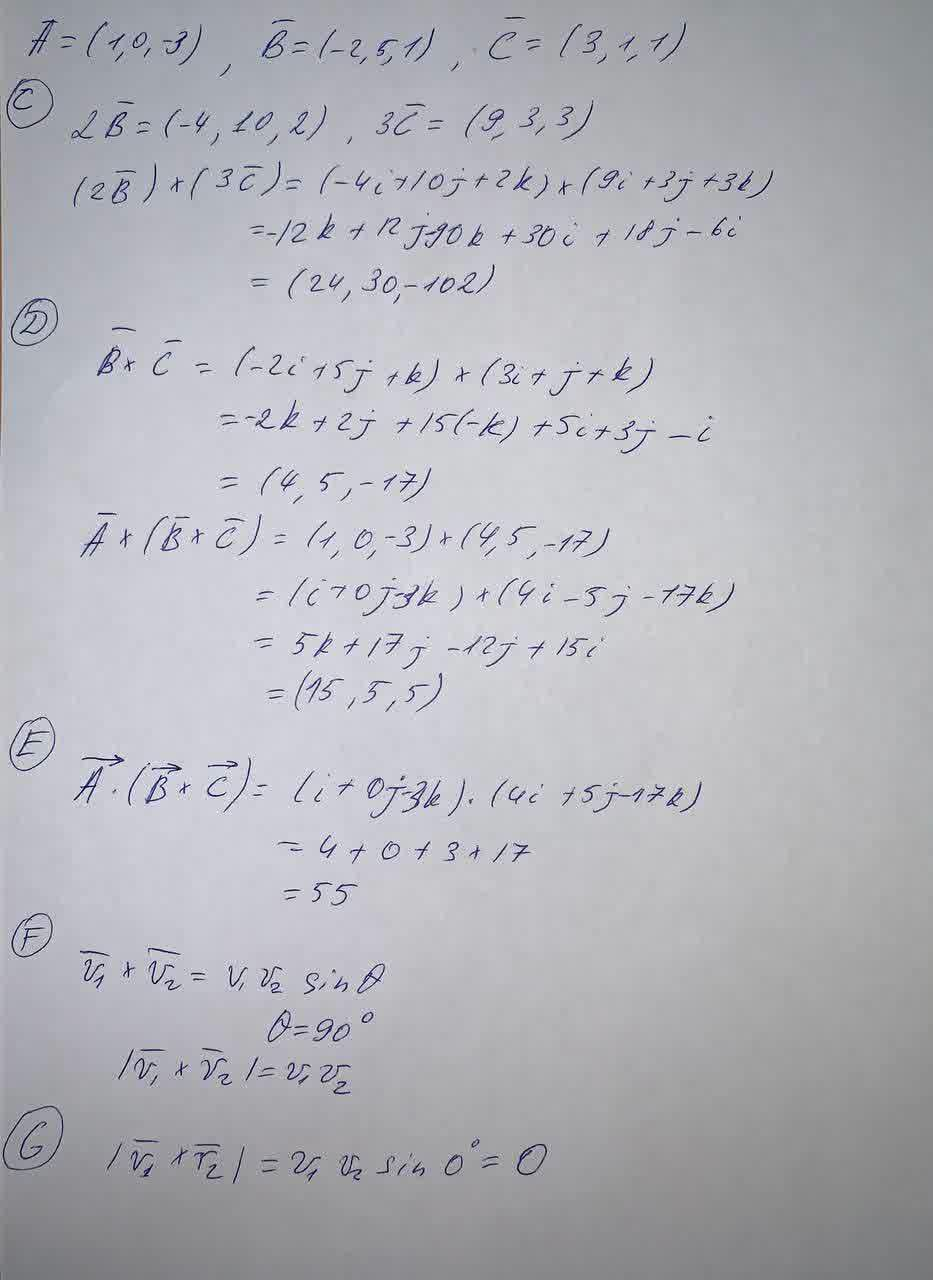Vector Cross Product. Let vectors A=(1,0,-3), B =(-2,5,1), and C =(3,1,1).Calculate the following, expressing your answers as ordered triplesKhaleesi Herbert 2021-09-20 Answered
Vector Cross Product
Let vectors A=(1,0,-3), B =(-2,5,1), and C =(3,1,1). Calculate the following, expressing your answers as ordered triples (three comma-separated numbers).
(C) $$\displaystyle{\left({2}\overline{{B}}\right)}{\left({3}\overline{{C}}\right)}$$
(D) $$\displaystyle{\left(\overline{{B}}\right)}{\left(\overline{{C}}\right)}$$
(E) $$\displaystyle{o}{v}{e}{r}\rightarrow{A}{\left({o}{v}{e}{r}\rightarrow{B}\times{o}{v}{e}{r}\rightarrow{C}\right)}$$
(F)If $$\displaystyle\overline{{v}}_{{1}}\ \text{ and }\ \overline{{v}}_{{2}}$$ are perpendicular, $$\displaystyle{\left|\overline{{v}}_{{1}}\times\overline{{v}}_{{2}}\right|}$$
(G) If $$\displaystyle\overline{{v}}_{{1}}\ \text{ and }\ \overline{{v}}_{{2}}$$ are parallel, $$\displaystyle{\left|\overline{{v}}_{{1}}\times\overline{{v}}_{{2}}\right|}$$

• Questions are typically answered in as fast as 30 minutes

Solve your problem for the price of one coffee

• Math expert for every subject
• Pay only if we can solve itViktor Wiley
Calculation: